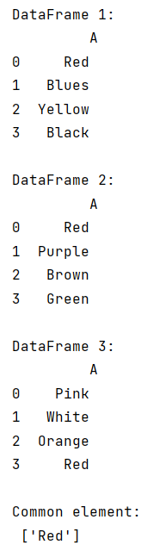# How to find common element or elements in multiple DataFrames?

Given multiple Pandas DataFrames, we have to find common element or elements in them.
Submitted by Pranit Sharma, on June 27, 2022

Pandas is a special tool that allows us to perform complex manipulations of data effectively and efficiently. Inside pandas, we mainly deal with a dataset in the form of DataFrame. DataFrames are 2-dimensional data structures in pandas. DataFrames consists of rows, columns, and the data.

While analyzing a Data Set, or while working on some new model, we sometimes have multiple DataFrames which may or may not contain similar values and even similar columns. We need to identify these values.

## Find common element or elements in multiple DataFrames

To find the common element or elements in multiple DataFrames, we will use reduce() method for a set and we will use the set.intersection() inside it so that all the duplicates will be removed and finally we will store the values in a list.

To work with pandas, we need to import pandas package first, below is the syntax:

```import pandas as pd
```

Let us understand with the help of an example,

## Python program to find common element or elements in multiple DataFrames

```# Importing pandas package
import pandas as pd

# Importing reduce function
from functools import reduce

# Creating  DataFrames
df1 = pd.DataFrame({'A':['Red','Blues','Yellow','Black']})
df2 = pd.DataFrame({'A':['Red','Purple','Brown','Green']})
df3 = pd.DataFrame({'A':['Pink','White','Orange','Red']})

# Display all the DataFrames
print("DataFrame 1:\n",df1,"\n")
print("DataFrame 2:\n",df2,"\n")
print("DataFrame 3:\n",df3,"\n")

# Finding a common element
result = list(reduce(set.intersection, map(set, [df1.A, df2.A, df3.A])))

# Display Result
print("Common element:\n",result)
```

## Output

The output of the above program is: